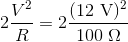# College Physics : Power

## Example Questions

### Example Question #1 : Circuits

A resistive heating element can be modeled as a resistor connected across the terminals of a battery.

If the battery is selected to be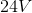, what should the resistance of the heating element be if the radiated power is to total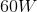?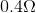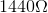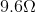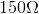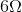Explanation:

We are asked to calculate the power dissipated through a resistor connected to a battery. We know that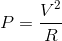. Substituting the values given above,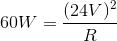. Solving for R yields.

### Example Question #1 : Power

What is the RMS voltage of a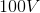peak-to-peak AC current?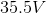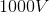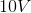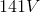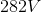Explanation:

This question requires two steps: the first is to calculate the peak voltage of the AC current, and the second requires conversion of the peak voltage to an RMS voltage. We are told that the current ispeak-to-peak. Thus, the peak voltage is half of this, or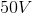. By definition, to convert peak voltage to RMS voltage, we must divide the peak voltage by: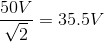### Example Question #21 : Electromagnetics

A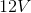battery is connected to two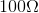light bulbs that are arrange in parallel. What is the total power dissipated by the light bulbs?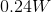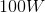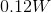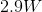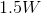The power dissipated in each light bulb is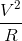. Since there are two light bulbs, the total power dissipated is